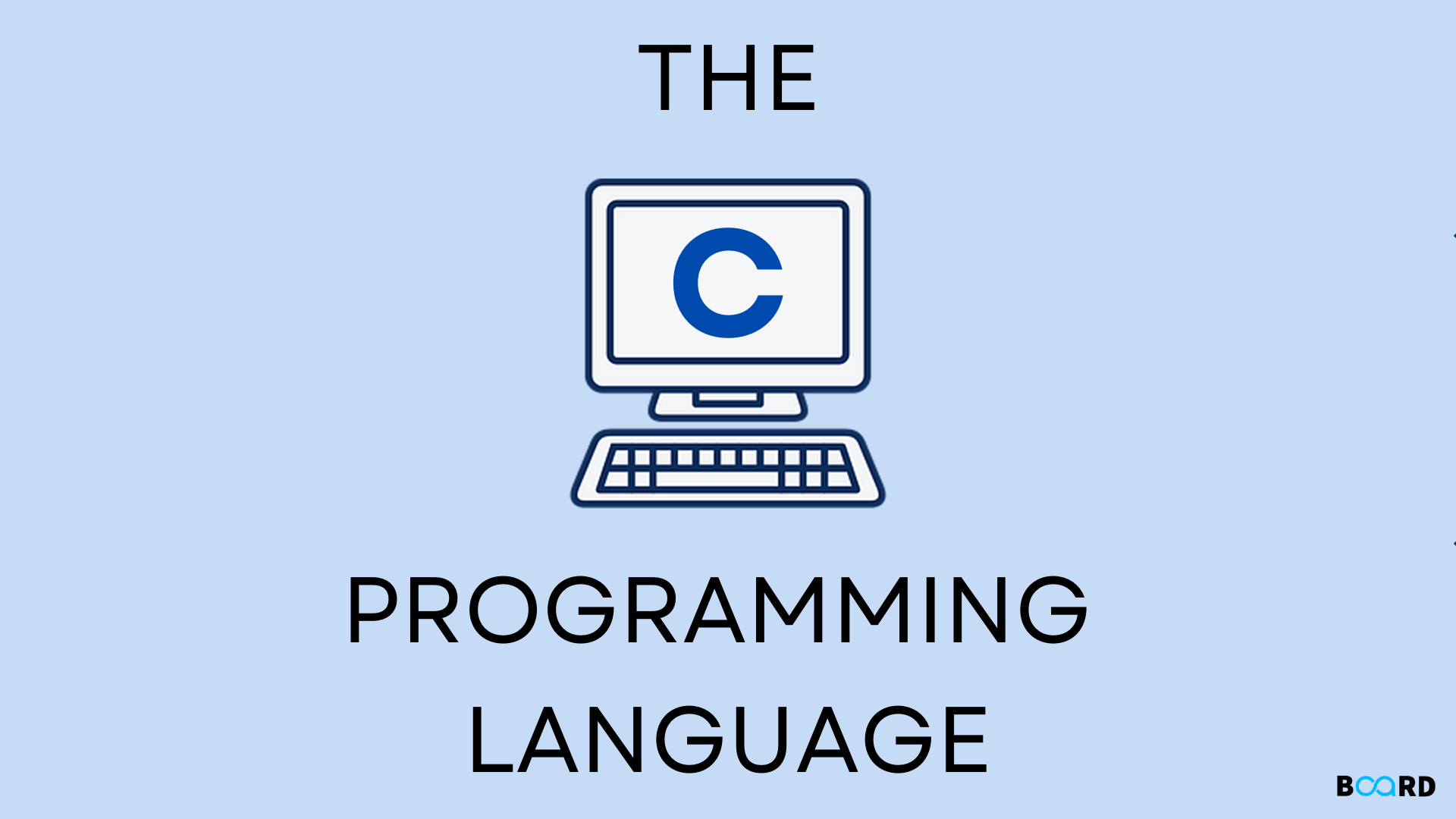# All about C Programming Language## Introduction

C is one of the most commonly used programming language.C is a procedural programming language.Dennis Ritchi developed this language to write program for operating system.C is also used in compiler development.

Now you must be thinking that why you should learn C Programming. Here are the reasons:

• C helps you to understand the internal architecture of a computer, how computer stores and retrieves information.
• After learning C, it will be much easier to learn other programming languages like Java, Python, etc.
• Opportunity to work on open source projects. Some of the largest open-source projects such as Linux kernel, Python interpreter, SQLite database, etc. are written in C programming.

## Keywords in C

Keywords are predefined, reserved words used in programming that have special meanings to the compiler. Keywords are part of the syntax and they cannot be used as an identifier.

A list of 32 keywords in the c language is given below:

 auto break case char const continue default do double else enum extern float for goto if int long register return short signed sizeof static struct switch typedef union unsigned void volatile while

## Identifiers in C

Identifier refers to name given to entities such as variables, functions, structures etc.Identifiers must be unique. They are created to give a unique name to an entity to identify it during the execution of the program.

int n = 10;

Here n is the identifier.

Let’s see some programs in C to understand this language better:

## C Program to find factorial of  a number

 #includeint find_factorial(int);int main(){   int num, fact;   //Ask user for the input and store it in num   printf("\nEnter any integer number:");   scanf("%d",&num);   //Calling our user defined function   fact =find_factorial(num);   //Displaying factorial of input number   printf("\nfactorial of %d is: %d",num, fact);   return 0;}int find_factorial(int n){   //Factorial of 0 is 1   if(n==0)      return(1);   //Function calling itself: recursion   return(n*find_factorial(n-1));}

Output:

 Enter any integer number: 4factorial of 4 is: 24
write your code here: Coding Playground

Let’s see a sorting algorithm in C Language:

## Insertion sort program in C

 #includeint main(){   /* Here i & j for loop counters, temp for swapping,    * count for total number of elements, number[] to    * store the input numbers in array. You can increase    * or decrease the size of number array as per requirement    */   int i, j, count, temp, number;   printf("How many numbers u are going to enter?: ");   scanf("%d",&count);   printf("Enter %d elements: ", count);   // This loop would store the input numbers in array   for(i=0;i=0)){         number[j+1]=number[j];         j=j-1;      }      number[j+1]=temp;   }   printf("Order of Sorted elements: ");   for(i=0;i

So this is how we write programs in C Language.Do visit our other blogs on Board Infinity to know more about C Language.Do share this blog and keep Learning.

write your code here: Coding Playground